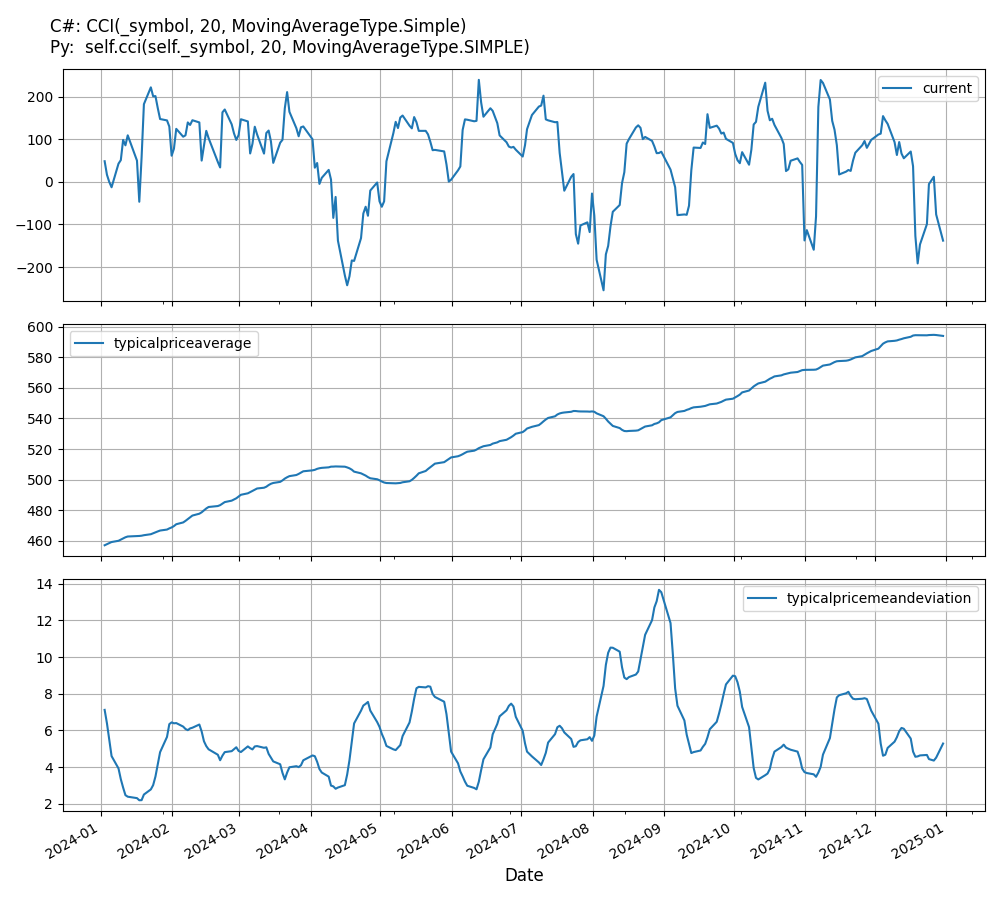# Supported Indicators

## Commodity Channel Index

### Introduction

This indicator represents the traditional commodity channel index (CCI) CCI = (Typical Price - 20-period SMA of TP) / (.015 * Mean Deviation) Typical Price (TP) = (High + Low + Close)/3 Constant = 0.015 There are four steps to calculating the Mean Deviation, first, subtract the most recent 20-period average of the typical price from each period's typical price. Second, take the absolute values of these numbers. Third, sum the absolute values. Fourth, divide by the total number of periods (20).

To view the implementation of this indicator, see the LEAN GitHub repository.

### Using CCI Indicator

To create an automatic indicators for CommodityChannelIndex, call the CCI helper method from the QCAlgorithm class. The CCI method creates a CommodityChannelIndex object, hooks it up for automatic updates, and returns it so you can used it in your algorithm. In most cases, you should call the helper method in the Initialize method.

public class CommodityChannelIndexAlgorithm : QCAlgorithm
{
private Symbol _symbol;
private CommodityChannelIndex _cci;

public override void Initialize()
{
_cci = CCI(_symbol, 20, MovingAverageType.Simple);
}

public override void OnData(Slice data)
{
{
// The current value of _cci is represented by itself (_cci)
// or _cci.Current.Value
Plot("CommodityChannelIndex", "cci", _cci);
// Plot all properties of cci
Plot("CommodityChannelIndex", "typicalpriceaverage", _cci.TypicalPriceAverage);
Plot("CommodityChannelIndex", "typicalpricemeandeviation", _cci.TypicalPriceMeanDeviation);
}
}
}
class CommodityChannelIndexAlgorithm(QCAlgorithm):
def Initialize(self) -> None:
self.cci = self.CCI(self.symbol, 20, MovingAverageType.Simple)

def OnData(self, slice: Slice) -> None:
# The current value of self.cci is represented by self.cci.Current.Value
self.Plot("CommodityChannelIndex", "cci", self.cci.Current.Value)
# Plot all attributes of self.cci
self.Plot("CommodityChannelIndex", "typicalpriceaverage", self.cci.TypicalPriceAverage.Current.Value)
self.Plot("CommodityChannelIndex", "typicalpricemeandeviation", self.cci.TypicalPriceMeanDeviation.Current.Value)


The following reference table describes the CCI method:

### CCI()1/1

            CommodityChannelIndex QuantConnect.Algorithm.QCAlgorithm.CCI (
Symbol                                symbol,
Int32                                 period,
*MovingAverageType                    movingAverageType,
*Nullable<Resolution>           resolution,
*Func<IBaseData, IBaseDataBar>  selector
)


Creates a new CommodityChannelIndex indicator. The indicator will be automatically updated on the given resolution.

If you don't provide a resolution, it defaults to the security resolution. If you provide a resolution, it must be greater than or equal to the resolution of the security. For instance, if you subscribe to hourly data for a security, you should update its indicator with data that spans 1 hour or longer.

The following table describes the MovingAverageType enumeration members:

You can manually create a CommodityChannelIndex indicator, so it doesn't automatically update. Manual indicators let you update their values with any data you choose.

Updating your indicator manually enables you to control when the indicator is updated and what data you use to update it. To manually update the indicator, call the Update method with a TradeBar, or QuoteBar. The indicator will only be ready after you prime it with enough data.

public class CommodityChannelIndexAlgorithm : QCAlgorithm
{
private Symbol _symbol;
private CommodityChannelIndex _cci;

public override void Initialize()
{
_cci = new CommodityChannelIndex(20, MovingAverageType.Simple);
}

public override void OnData(Slice data)
{
if (data.Bars.TryGeValue(_symbol, out var bar))
{
_cci.Update(bar);
}

{
// The current value of _cci is represented by itself (_cci)
// or _cci.Current.Value
Plot("CommodityChannelIndex", "cci", _cci);
// Plot all properties of cci
Plot("CommodityChannelIndex", "typicalpriceaverage", _cci.TypicalPriceAverage);
Plot("CommodityChannelIndex", "typicalpricemeandeviation", _cci.TypicalPriceMeanDeviation);
}
}
}
class CommodityChannelIndexAlgorithm(QCAlgorithm):
def Initialize(self) -> None:
self.cci = CommodityChannelIndex(20, MovingAverageType.Simple)

def OnData(self, slice: Slice) -> None:
bar = slice.Bars.get(self.symbol)
if bar:
self.cci.Update(bar)

# The current value of self.cci is represented by self.cci.Current.Value
self.Plot("CommodityChannelIndex", "cci", self.cci.Current.Value)
# Plot all attributes of self.cci
self.Plot("CommodityChannelIndex", "typicalpriceaverage", self.cci.TypicalPriceAverage.Current.Value)
self.Plot("CommodityChannelIndex", "typicalpricemeandeviation", self.cci.TypicalPriceMeanDeviation.Current.Value)


To register a manual indicator for automatic updates with the security data, call the RegisterIndicator method.

public class CommodityChannelIndexAlgorithm : QCAlgorithm
{
private Symbol _symbol;
private CommodityChannelIndex _cci;

public override void Initialize()
{
_cci = new CommodityChannelIndex(20, MovingAverageType.Simple);
RegisterIndicator(_symbol, _cci, Resolution.Daily);
}

public override void OnData(Slice data)
{
{
// The current value of _cci is represented by itself (_cci)
// or _cci.Current.Value
Plot("CommodityChannelIndex", "cci", _cci);
// Plot all properties of cci
Plot("CommodityChannelIndex", "typicalpriceaverage", _cci.TypicalPriceAverage);
Plot("CommodityChannelIndex", "typicalpricemeandeviation", _cci.TypicalPriceMeanDeviation);
}
}
}
class CommodityChannelIndexAlgorithm(QCAlgorithm):
def Initialize(self) -> None:
self.cci = CommodityChannelIndex(20, MovingAverageType.Simple)
self.RegisterIndicator(self.symbol, self.cci, Resolution.Daily)

def OnData(self, slice: Slice) -> None:
# The current value of self.cci is represented by self.cci.Current.Value
self.Plot("CommodityChannelIndex", "cci", self.cci.Current.Value)
# Plot all attributes of self.cci
self.Plot("CommodityChannelIndex", "typicalpriceaverage", self.cci.TypicalPriceAverage.Current.Value)
self.Plot("CommodityChannelIndex", "typicalpricemeandeviation", self.cci.TypicalPriceMeanDeviation.Current.Value)


The following reference table describes the CommodityChannelIndex constructor:

### CommodityChannelIndex()1/2

            CommodityChannelIndex QuantConnect.Indicators.CommodityChannelIndex (
int                 period,
*MovingAverageType  movingAverageType
)


Initializes a new instance of the CommodityChannelIndex class.

### CommodityChannelIndex()2/2

            CommodityChannelIndex QuantConnect.Indicators.CommodityChannelIndex (
string              name,
int                 period,
*MovingAverageType  movingAverageType
)


Initializes a new instance of the CommodityChannelIndex class.

### Visualization

The following image shows plot values of selected properties of CommodityChannelIndex using the plotly library.You can also see our Videos. You can also get in touch with us via Discord.